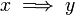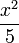# User talk:Emanuele

(Difference between revisions)
 Revision as of 22:12, 14 January 2010 (view source)Emanuele (Talk | contribs)← Older edit Revision as of 22:19, 14 January 2010 (view source)Emanuele (Talk | contribs) Newer edit → Line 1: Line 1: testing math equations testing math equations + $x \implies y$ $\frac{x^2}{5}$ $\frac{x^2}{5}$

## Revision as of 22:19, 14 January 2010

testing math equations$x \implies y$$\frac{x^2}{5}$